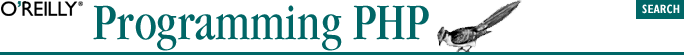home | O'Reilly's CD bookshelfs | FreeBSD | Linux | Cisco | Cisco Exam## 14.13. Resources

Use resources in your extensions when the extension is providing an interface to something that needs cleanup. When the resource goes out of scope or your script ends, your destructor function for that resource is called, and you can free memory, close network connections, remove temporary files, etc.

```static int le_test;

typedef struct _test_le_struct {
char *name;
long age;
} test_le_struct;```
```static void _php_free_test(zend_rsrc_list_entry *rsrc TSRMLS_DC) {
test_le_struct *test_struct = (test_le_struct *)rsrc->ptr;

efree(test_struct->name);
efree(test_struct);
}```
```le_test = zend_register_list_destructors_ex(_php_free_test, NULL, "test",
module_number);```

Now, here's a fictitious my_init( ) function that initializes the data associated with the resource. It takes a string and an integer (name and age):

```PHP_FUNCTION(my_init) {
char *name = NULL;
int name_len, age;
test_le_struct *test_struct;

if (zend_parse_parameters(ZEND_NUM_ARGS( ) TSRMLS_CC, "sl", &name,
&name_len, &age) == FAILURE) {
return;
}
test_struct = emalloc(sizeof(test_le_struct));
test_struct->name = estrndup(name, name_len);
test_struct->age = age;
ZEND_REGISTER_RESOURCE(return_value, test_struct, le_test);
}```

And here's a my_get( ) function that takes a resource parameter returned from my_init( ) and uses that to look up the data associated with the resource:

```PHP_FUNCTION(my_get)
{
test_le_struct *test_struct;
zval *res;

if (zend_parse_parameters(ZEND_NUM_ARGS( ) TSRMLS_CC, "r", &res)
== FAILURE) {
return;
}

ZEND_FETCH_RESOURCE(test_struct, test_le_struct *, &res, -1, "test",
le_test);

if(!test_struct) RETURN_FALSE;

array_init(return_value);